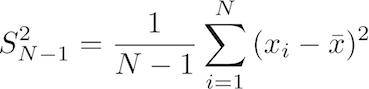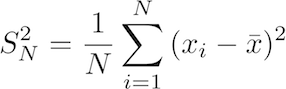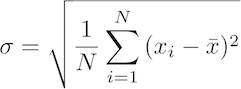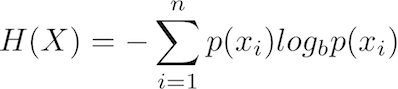Translate equations to R
by Damir Cavar (March 2010)

In this section we will take a look at how to translate some typical equation into R. Let us start with the formula for variance:The LaTeX code for the equation is: S^2_{N-1}=\frac{1}{N-1}\sum_{i=1}^N{(x_i - \bar{x})^2}

What this formula says is the following:

a. Given a vector of values (some experimental results or measures) of length N, for example:

x <- c(1, 2, 4, 5, 6, 8, 9)

In this case, x is of length 7, that is N = 7, which can be verified by using the following function in R:

length(x)

b. for each value, from the beginning (index position i = 1), to the last element (index position i = N = 7),
subtract the arithmetic mean of the distribution from this value, and square the result:

The arithmetic mean of the distribution can be calculated using:

mean(x)

The subtraction and squaring can be calculated as:

(x - mean(x))^2

The resulting vector for x as defined above should look like:

16 9 1 0 1 9 16

c. sum up all the resulting values, and divide the result by (N - 1).

Summing up the values in a list can be achieved by using the function sum():

sum(x)

The output for x as defined above should be:

35

To complete our calculation using steps a. and b., the formula so far should be translated as:

sum( (x - mean(x))^2 )

The output of this calculation for the defined x should be:

52

The final step is to divide this part of the calculation result by N, which is the length of x reduced by 1:

sum( (x - mean(x))^2 ) / (length(x) - 1)

The resulting value:

8.666667

is the variance of the measures in x, as defined above.

We can define this calculation as a function, and store it in some external file for reuse. A function can be defined in the following way:

s2variance <- function (x) { sum( (x - mean(x))^2 ) / (length(x) - 1) }

s2variance is now defined as a function that takes one parameter, i.e. a vector of values, measures or results. The returned value is the variance of the distribution, as explained above. For example:

s2variance( c(3, 5, 1, 1, 2, 1, 0, 4) )

should return the value:

2.982143

In fact, R comes with a predefined function for variance calculation, as explained in the previous section (the function var), such that:

var( c(3, 5, 1, 1, 2, 1, 0, 4) )

should return the same value as our own function definition above, i.e.:

2.982143

A variation of this equation is the variance of a population:The LaTeX code for this equation is: S^2_N=\frac{1}{N}\sum_{i=1}^N{(x_i - \bar{x})^2}

The only difference is that in this function we do not divide by the length of x minus 1, but just by the length of x, as in the following R code:

sum( (x - mean(x))^2 ) / length(x)

Let us consider now the equation for the Standard deviation:The LaTeX code for this equation is: \sigma = \sqrt{ \frac{1}{N} \sum_{i=1}^N{ (x_i - \bar{x})^2 }}

This equation is just the square root of the population variance. The corresponding R code is:

sqrt( sum( (x - mean(x))^2 ) / length(x) )

To declare this code as a function for storage and reuse, just wrap it in a function declaration as:

sdd <- function (x) { sqrt( sum( (x - mean(x))^2 ) / length(x) ) }

and use it as follows:

sdd(x)

which should return:

2.725541

Let us consider a random variable X, represented here as a vector with probabilities:

x <- c(0.1, 0.3, 0.15, 0.25, 0.01, 0.08, 0.01, 0.06, 0.04)

The vector x in this case is a complete list of event probabilities, such that the sum of all probabilities in x is equal to 1, as can be verified with the following R code:

sum(x)

Now, the (Shannon) entropy H(x) is defined as:The LaTeX code for this equation is: H(X) = -\sum_{i=1}^n{p(x_i)log_b p(x_i)}

The equation says that all probabilities of events for the random variable X have to be multiplied with the logarithm of them, summarized and multiplied by -1. We use here the logarithm to the base of 2, which is in R:

log2(x)

Thus, given that the vector x contains probabilities, the multiplication of each single probability with the log to the base of two of it, is in R:

x * log2(x)

For x as defined above, the resulting list should look like:

-0.33219281 -0.52108968 -0.41054484 -0.50000000 -0.06643856 -0.29150850 -0.06643856 -0.24353362 -0.18575425

Summing up the values in the resulting list can be achieved by the function sum:

sum(x * log2(x))

and the absolute value of this summation is returned by multiplying with -1:

-sum(x * log2(x))

By the way, the initial sum is always negative, because the log of a probability is always negative, given that a probability is between 0 and 1.